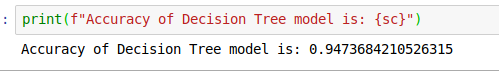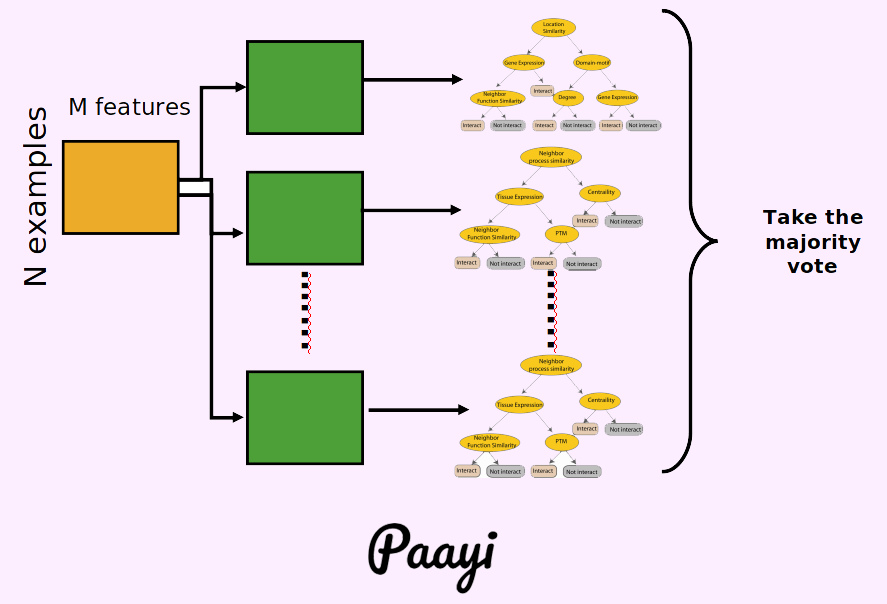## Python Tutorials: A Quick Guide to Decision Tree and Random Forest Algorithms in Python

##### Python Machine Learning: Learn Decision Tree & Random Forest Algorithms
Written by |20-Oct-2020 | 0 Comments | 377 Views

Now we will apply the same iris data set on the decision tree algorithm. Following is the code to implement.

 from sklearn import tree from sklearn.datasets import load_iris from sklearn.cross_validation import train_test_split from sklearn import metrics

Same as we have done before we first import the modules.

 data = load_iris() X = data.data y = data.target X_train,X_test,y_train,y_test = train_test_split(X,y,test_size=0.25,random_state=5)

Now we have split the data into train and test data

 model = tree.DecisionTreeClassifier(criterion='gini') model.fit(X_train,y_train) pred = model.predict(X_test) sc = metrics.accuracy_score(y_test,pred) print(f"Accuracy of Decision Tree model is: {sc}")

Now we have trained the model. The accuracy is low as compared to KNN. The decision tree is not the right choice for critical works. We have to use its variations, which beat almost all the machine learning models.

The output of the above is as follows:Figure 1

Random Forest

Random forest is an ensemble classifier that consists of many decision trees and outputs the class that is part of the classes of distinct trees. The term came from the random decision. The term came from random decision forests that were first proposed by Tin Kam Ho of Bell Labs in 1995. The method combines Breiman's "Bagging" idea and the random selection of features.

A random forest is a tool that leverages the power of many decision trees, judicious randomization, and ensemble learning to produce astonishingly accurate predictive models, insightful variable importance rankings, missing value imputations, novel segmentation, and laser-sharp reporting on a record by record basis for deep data understanding.

Essential Aspects of Random Forest:

• Random Forest are collections of a different decision tree that by bootstrapping technique
• The basic unit of random forest is CART from where it is inspired.
• It draws out the random sample from your main database and builds a decision tree on this random sample.
• The sample would use half of the available data, although it could be a different fraction.

Bagging:

Bagging is the combination of two words "Bootstrap" and "Aggregation." Is used when our goal is to reduce the variance of the decision tree. The idea of bagging is to create several subsets of data from the training sample chosen randomly with replacement.

Now each collection of subset data is used to train their decision trees. As a result, we end up with an ensemble of different models. Average of all the predictions from different trees are used, which is more robust than a single decision tree, which is called aggregation.

Random forest is an extension of over bagging. It takes one extra step where, in addition to taking the random dataset, it also takes the random selection of features to grow trees when you have many random trees.

Random Forest Algorithm:

• First, it draws bootstrap sample Z* of size N from the training data.
• Random forest tree grows to the bootstrapped data by recursive action until the minimum node nmin is reached.
>> Select the random variables from all the variables.
>> Pick the best variable by calculating feature importance.
>>Split the node into two daughter nodes.
• Output the ensemble tree.
• Predict from unknown features and select with majority voting.

Difference to Standard Tree:

• Train each tree on bootstrap re-sample of data
• For each split, consider only a few randomly selected variables.
• No pruning occurs in a random forest.
• Fit trees in such a way and use average or majority voting to aggregate results.

How randomness should a random forest have?

At one extreme, if we pick every splitter at random, we obtain randomness everywhere in the tree. Usually, this does not perform very well. A less extreme method is to first select a subset of candidate predictors at random and then produce the split by selecting the best splitter available.

If we have 1000 predictors, we might select a random set of 30 in each node and then split using the best predictor among the 30 available instead of the best among the full 1000.

Mostly it is assumed that we select a random subset of predictor once and start analysis and then grow the tree. However, this is not the case; we select a subset at every node of a tree.

How many predictors in a node?

Some values advised by Brieman and Cutler:

Following are the rules:

If we have n predictors, than the following are the suggested possible rules:

 sqrt( n ) 5 sqrt ( n ) 2 * sqrt( n ) ln2( n )

Working of Random Forest:

We have seen a decision tree which looks like this:Each node is split based on impurity present in the database. To convert such type of decision tree into a random forest decision tree, we have to make many decision trees in which predictors are taken randomly. Visually we can represent it as:As the number of trees grows, the accuracy will be increased.

Voting System:

Each tree will then give its output. The outputs are then calculated. The output, which is in maximum number, will be considered the true output, and this process is called a voting system.

Voting is further divided into the following categories:

​​​​​​​Majority Voting:

Each model makes a forecast for each test occurrence, and the last yield expectation is the one that gets the more significant part of the votes. If none of the expectations get the more significant part of the votes, we may state that the group strategy couldn't make a steady forecast for this example. Even though this is generally utilized the system, you may attempt the most cast a ballot forecast as the last expectation.

​​​​​​​Weighted Voting:

Unlike the majority voting,  where each model has similar rights, we can expand the significance of at least one demonstrates. In weighted casting a ballot, you check the forecast of the better model on various occasions. Finding a sensible arrangement of loads is up to you.

​​​​​​​Simple Averaging:

In a simple averaging method, for every row of test data set, the average predictions are calculated. This method often reduces over fit and creates a smoother regression model.

​​​​​​​Weighted Averaging:

The weighted average is a slightly modified version of simple averaging, where the prediction of each model is multiplied by the weight, and then their average is calculated.